# Test: Previous Year Questions - Isomerism (Level 1)

## 17 Questions MCQ Test Chemistry Class 11 | Test: Previous Year Questions - Isomerism (Level 1)

Description
This mock test of Test: Previous Year Questions - Isomerism (Level 1) for Class 11 helps you for every Class 11 entrance exam. This contains 17 Multiple Choice Questions for Class 11 Test: Previous Year Questions - Isomerism (Level 1) (mcq) to study with solutions a complete question bank. The solved questions answers in this Test: Previous Year Questions - Isomerism (Level 1) quiz give you a good mix of easy questions and tough questions. Class 11 students definitely take this Test: Previous Year Questions - Isomerism (Level 1) exercise for a better result in the exam. You can find other Test: Previous Year Questions - Isomerism (Level 1) extra questions, long questions & short questions for Class 11 on EduRev as well by searching above.
QUESTION: 1

### Stereo - Isomerism includes -  [AIEEE - 2002]

Solution:

Stereoisomerism is defined as two molecules which have the same molecular formula but the atoms are arranged differently in space. The other major form of stereoisomerism is optical isomerism. Optical isomers arise when a chiral carbon is present, this is a carbon atom attached to four different groups.

QUESTION: 2

### Which of the following does not show geometrical isomerism -  [AIEEE-2002]

Solution:

The correct answer is Option B.
Conditions of geometrical isomerism :
(I) Geometrical isomerism arises due to the presence of a double bond or a ring structure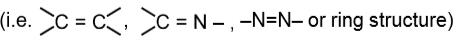Due to the rigidity of double bonds or the ring structure the molecules exist in two or more orientations. This rigidity to rotation is described as restricted rotation / hindered rotation / no rotation.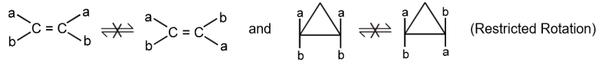(II) Different groups should be attached at each doubly bonded atom.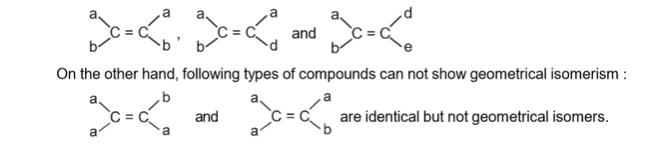(III) Groups responsible to show geometrical isomerism must be nearly in the same plane.

CH3CH2CH=CH2 , can’t show geometric isomerism because it does not satisfy the above condition.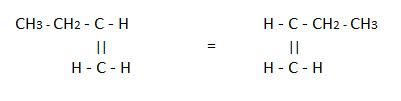Both are the same,

QUESTION: 3

### Racemic mixture is formed by mixing two  -   [AIEEE-2002]

Solution:

A racemic mixture consists of chiral molecules, but it has no net optical activity. The process by which a racemic mixture is formed from chiral materials is called racemization. One way to do this is to mix equal amounts of enantiomeric substance.

QUESTION: 4

Geometrical isomerism is not shown by -

[AIEEE-2002]

Solution:

The correct answer is option A
From all the compounds in the question only  1,1-dichloro-1-pentene has no geometrical isomer(no cis-trans).’cis’ form or ‘trans’ form occour when some groups placed in two different doubly bonded carbon in same side (cis form) or in opposite side (trans form).
Here two ‘Cl’ groups present in one carbon. Hence here is no possibility to form any geometrical isomer.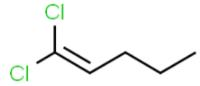QUESTION: 5

Racemic mixture is -

[AIEEE-2002]

Solution:

The correct answer is option A
Chiral carbon atoms are also referred to as 'stereogenic carbons' or 'asymmetrical carbon atoms'. Compound 1 has a chiral carbon center, because it is attached to four different groups (W, X, Y and Z).

QUESTION: 6

Among the following four structures I to IV

(i)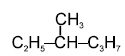(ii)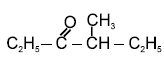(iii)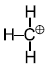(iv)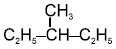It is true that -

[AIEEE-2003]

Solution:

Chiral compounds are those which have one chiral centre. All four atoms or groups attached to carbon are different.
Only (i) and (ii) are chiral carbons.

QUESTION: 7

Which of the following compounds is not chiral ?

[AIEEE-2004]

Solution:

The correct answer is option A
1-chloro-pentane
CH3−CH2−CH2−CH2−CH2Cl
As it does not contain a chiral centre so it is optically inactive (not chiral)compound.

QUESTION: 8

Which of the following will have a mesoisomer also -

[AIEEE-2004]

Solution: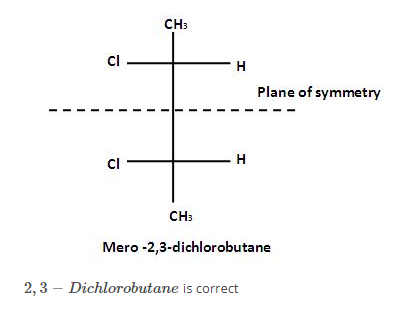QUESTION: 9

Which types of isomerism is shown by 2,3 - dichlorobutane ?

[AIEEE-2005]

Solution: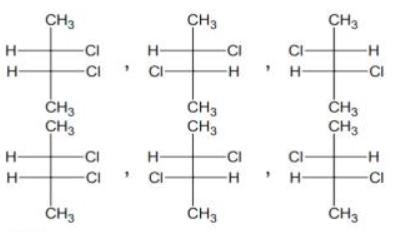QUESTION: 10

Increasing order of stability among the three main conformations (i.e. Eclipse, Anti, Gauche) of 2 - fluoroethanol is

[AIEEE 2006]

Solution: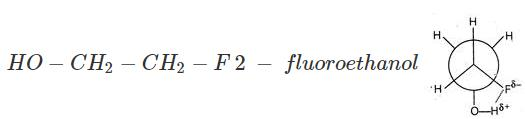Gauche conformation is comparatively more stable due to hydrogen linkage in between F and H (atO−atom), hence order is Eclipse, Anti (staggered), Gauche.

*Multiple options can be correct
QUESTION: 11

Which of the following molecules is expected to rotate the plane of plane-polarised light?

[AIEEE-2007]

Solution:

For A :The molecule, which rs optically active, has chiral centre, is expected to rotate the plane of polarised light.
For C :Two chiral centres, but plane of symmetry within molecule ⇒ optically inactive.
For D: Molecule has chiral centre is expected to rotate plane polarised light.
Hence,option A and D is correct.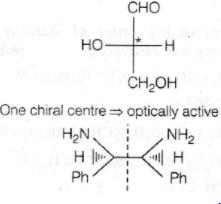QUESTION: 12

Which one of the following conformations of cyclohexane is chiral ?

[AIEEE-2007]

Solution:

Twist boat form is chiral as it lacks plane of symmetry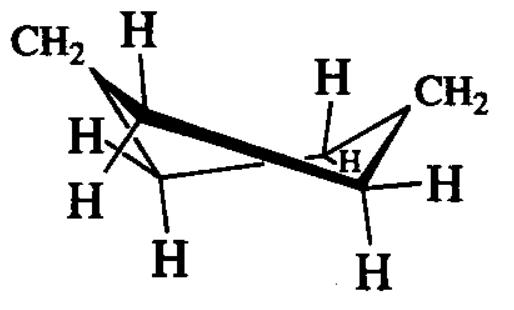QUESTION: 13

The absolute configuration of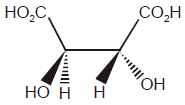is

[AIEEE-2008]

Solution:

Four different groups attached to chiral center are arranged in decreasing order of priority. The lowest priority group is placed below the plane of paper. When the remaining 3 groups in decreasing order of priority are in clockwise direction, the configuration is R and when the direction is counterclockwise, the configuration is S.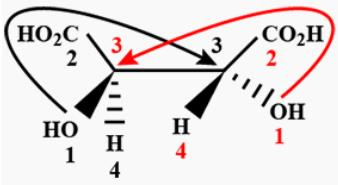QUESTION: 14

The alkene that exhibits geometrical isomerism is :

[AIEEE-2009]

Solution:

The alkene that exhibits geometrical isomerism is. 2-Butene may exist as cis and trans isomers. The cis-isomer has the two methyl groups on the same side and the trans-isomer has the two methyl groups on opposite sides. Due to restricted rotation around double bond it exhibits geometrical isomerism.

QUESTION: 15

The number of stereoisomers possible for a compound of the molecular formula

CH3 - CH = CH - CH(OH) - Me  is :

[AIEEE-2009]

Solution:

About the double bond, two geometrical isomers are possible and the compound is having one chiral carbon.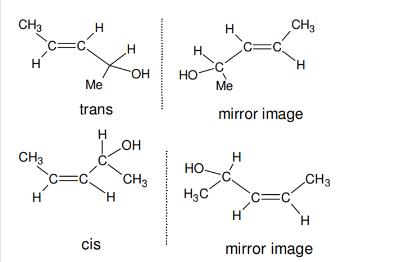QUESTION: 16

Out of the following, the alkene that exhibits optical isomerism is

[AIEEE-2010]

Solution: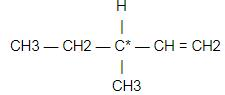The correct answer is 3-methyl-1-pentene because it has one chiral centre.

QUESTION: 17

How many chiral compounds are possible on monochlorination of 2 - methyl butane?

[AIEEE-2012]

Solution:

The correct answer is option A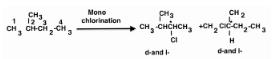Chlorination at C-2 and C-4 produces no chiral compound.Hence (A) is the correct answer.